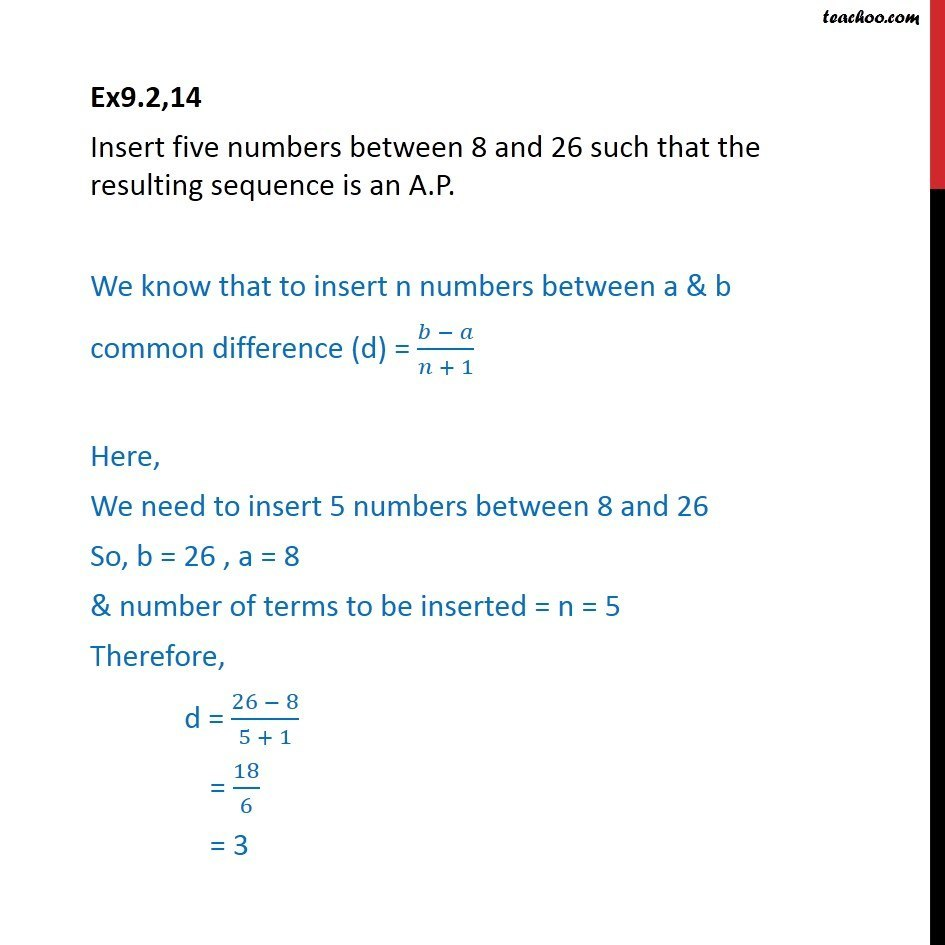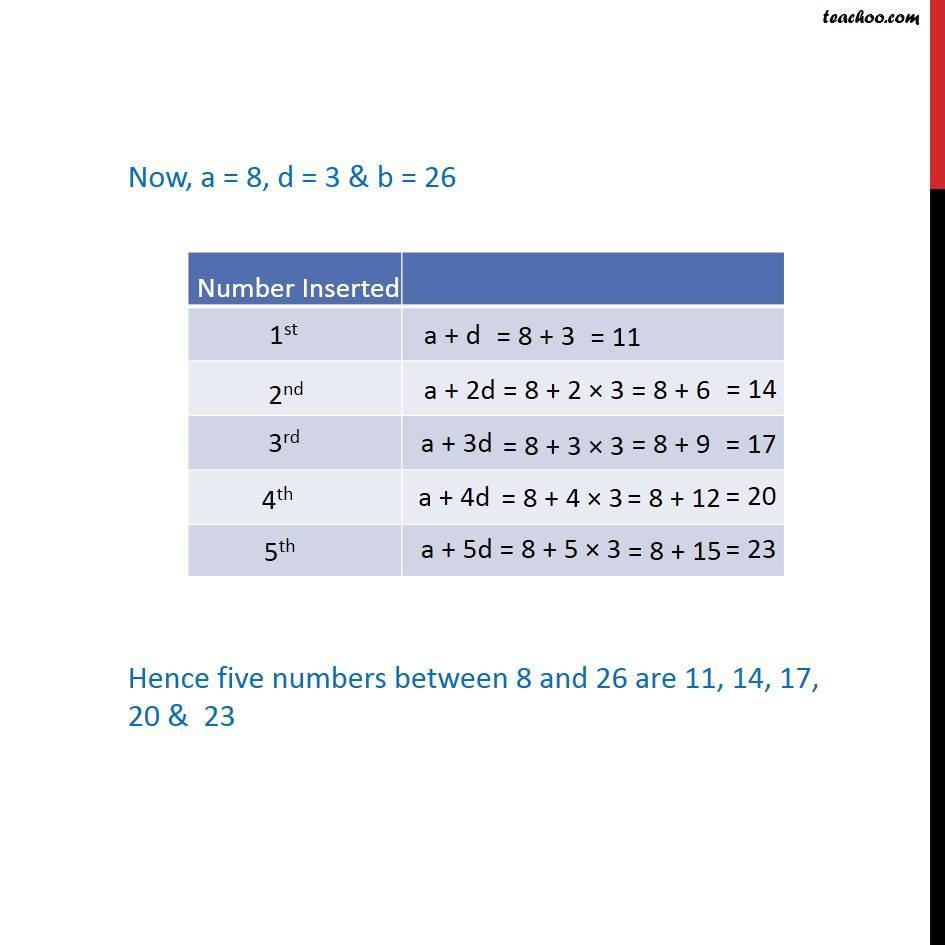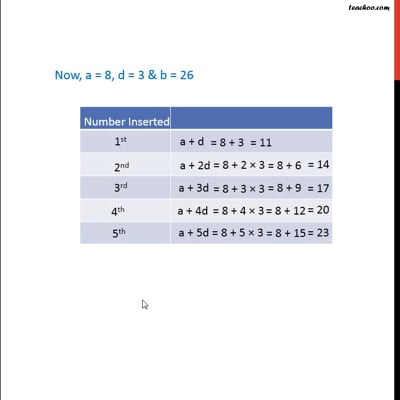Ex 9.2

Chapter 9 Class 11 Sequences and Series
Serial order wiseThis video is only available for Teachoo black users

Introducing your new favourite teacher - Teachoo Black, at only ₹83 per month

### Transcript

Ex9.2,14 Insert five numbers between 8 and 26 such that the resulting sequence is an A.P. We know that to insert n numbers between a & b common difference (d) = ( )/( + 1) Here, We need to insert 5 numbers between 8 and 26 So, b = 26 , a = 8 & number of terms to be inserted = n = 5 Therefore, d = (26 8)/(5 + 1) = 18/6 = 3 Now, a = 8, d = 3 & b = 26 Hence five numbers between 8 and 26 are 11, 14, 17, 20 & 23